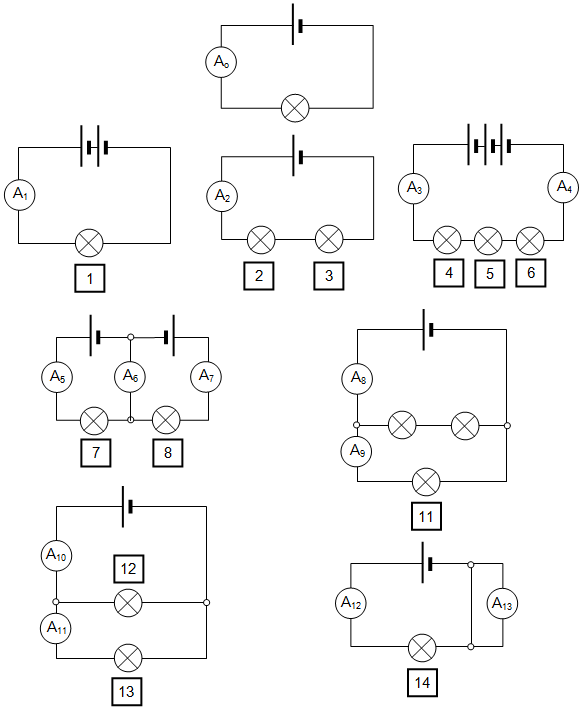## Electricity

Where needed take the charge on the electron to be –1.6x10-19 C

1. If the current through the ammeter in the first circuit (Ao) with one cell and one bulb is 0.2 A calculate the expected currents in all the other ammeters assuming that all the cells and bulbs are identical.2. What will be the reading on ammeter 6 if bulb 8 blows?

3. What will be the reading on ammeter 7 if bulb 8 blows?

4. What will be the reading on ammeter 9 if bulb 10 blows?

5. What will be the reading on ammeter 10 if bulb 12 blows?

6. What will be the reading on ammeter 11 if bulb 13 blows?

7. If a current of 25 mA flows in a wire how many electrons pass that point per second?

8. Define:
(a) resistance
(b) resistivity

9. Draw a graph to show how the current varies with the voltage for:
(a) a metal filament in a light bulb
(b) a semiconductor

10. Calculate:
(a) the current that flows in a resistor of 200Ω when connected to a voltage of 240 V
(b) the current that flows in a resistor of 50Ω when connected to a voltage of 30 V
(c) the voltage across a 400Ω resistor when a current of 20 mA flow through it
(d) the resistance of a 25 cm long metal wire that has a resistivity of 2.5x10-8 Ωm and a diameter of 0.2 mm.